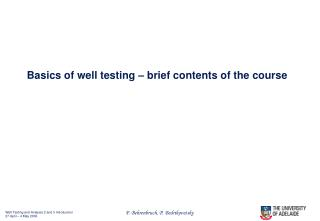# Basics of well testing – brief contents of the course - PowerPoint PPT PresentationDownload PresentationBasics of well testing – brief contents of the course

Basics of well testing – brief contents of the course
Download Presentation## Basics of well testing – brief contents of the course

- - - - - - - - - - - - - - - - - - - - - - - - - - - E N D - - - - - - - - - - - - - - - - - - - - - - - - - - -
##### Presentation Transcript

1. Basics of well testing – brief contents of the course

2. One-dimensional flow of liquid:The mass conservation law φρ ρ u ρ u x + Δ x x Change of mass in volume = Flow in – Flow out per time unit VolumeV: cylinder with cross-section A limited by the planes x and x + dx. Mass density: Flow in minus flow out: Derivation of the differential conservation law and tend to zero: Divide by

3. Q. Derive the mass conservation law for the tube with the variable cross-section: Q. Using the result of the previous problem, derive the mass conservation law for the radial influx:

4. Answers Mass conservation in the tube of variable cross-section: Mass conservation for the radial inflow:

5. General mass conservation law in three dimensions: Q: Obtain axi symmetric mass balance equation p=p(r,t) using cylindrical co-ordinates in 3d equation:

6. Basic idea of well testing • The well tests consist of relatively fast opening/closing wells, measuring the flowrates and following the value of the well bore pressure – non-steady state • Practical well testing is carried out with the help of a special software • The software is based on simple solutions of the flow equations Continuity (radial inflow): Pressure conductivity (or Pressure diffusivity or Piesoconductivity) equation: Darcy:

7. Fluid compressibility coefficient(do not mix with gas compressibility Z(p)!) Q. Determine units (dimension) of fluid compressibility coefficient Q. Evaluate the compressibility k-t of ideal gas under characteristic reservoir pressures. Do you believe that the compressibility concept is applicable to gas well testing? Q. Will the real gas and the liquid compressibilities be higher or lower than that of ideal gas? Order of magnitude for c = 10-4-10-5 1/bar for oil and c = 10-6 1/bar for water

8. in the last equation may be treated as constants Pressure conductivity equation in terms of compressibility:

9. Effects of fluid and rock compressibility Overburden pressure: the reservoir “keeps” the upper rock column Porosity becomes a pressure function Definition of formation compressibility cf = 10-3 – 10-5 1/bar From now on c is a total of the fluid and formation compressibilities

10. Dimensional analysis Reduction of dimensions:

11. Pressure diffusivity coefficient (L2/T) Evaluation of maximum and minimum pressure diffusivity

12. Q. Determine dimention of D Q. Evaluate the value of D for the cases of a) well exploitation (t0 = years) and b) well testing (hours). Use the following parameters: Permeability a) 0.1d, b) 1md; Time t0 a) 2 years, b) 1 hour; Compressibility factor 10-10; oil viscosity 3 cP; porosity 0.25; well radius 10cm.

13. Answer: On the times characteristic of reservoir development the time derivative may be neglected, and development occurs in the quasi-steady state regime. On the times characteristic of well testing the non-steady state character of the process becomes important.

14. Three regimes of production through isolated well 1. Spreading of perturbation around the well (hours) – to be used for well testing B.C. 2. Uniform decrease of the reservoir pressure – semi steady-state regime (days, months) B.C. 3. Slow decrease of the pressure drop, decrease of production (years) B.C.

15. Early stage: Constant terminal rate solution Initial and boundary conditions: Linearization for oil: Self-similarity: Neither equation, nor the boundary conditions change if the following substitution is made: Q. Check the condition of self-similarity.

16. Boltzmann substitution As a consequence of self-similarity, the solution depends on the only variable We have: So, the equation is transformed to the ordinary differential equation Transformation of the initial and boundary conditions:

17. Solution of the differential equation First substitution: First integration: From the boundary condition: Second integration:

18. Exponential integral Solution for pressure in terms of the exponential integral: Approximation of the exponential integral: Approximate solution, including skin-factor:

19. Pressure diffusivity coefficient (L2/T) Evaluation of maximum and minimum pressure diffusivity Arrival time of the pressure wave Evaluates: r=rw=0.1, r=10 m, r=re=333 m

20. Q. For times between 0 and 1 hour, and other parameters from the problem on slide 7 (with the only permeability of 0.1 Da), and for the skin factors equal to 0. and 1., build the plots of the dependence of pressure at the well versus time (use just 2 or 3 intermediate points). Q. May skin be negative? Q. In practical well testing, the derivative dP/dt is often used instead for P(t). Find this derivative and explain why it is so important.